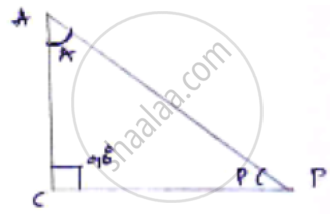Share
Notifications

View all notifications
Books Shortlist
Your shortlist is empty

# If ∠A and ∠P Are Acute Angles Such that Tan a = Tan P, Then Show that ∠A = ∠P. - CBSE Class 10 - Mathematics

Login
Create free account

Forgot password?
ConceptTrigonometric Ratios of an Acute Angle of a Right-angled Triangle

#### Question

If ∠A and ∠P are acute angles such that tan A = tan P, then show that ∠A = ∠P.

#### Solution

A and P are acute angle tan A = tan P

Let us consider right angled triangle ACP,We know tan theta = "opposite side"/"adjacent side"

tan A = (PC)/(AC)

tan A = (AC)/(PC)

tan A = (AC)/(PC)

tan =tan P

(DC)/(AC) = (AC)/(PC)

(PC)^2 = (AC)^2

𝑃𝐶 = 𝐴𝐶   [∵ Angle opposite to equal sides are equal]

∠𝑃 = ∠𝐴

Is there an error in this question or solution?

#### APPEARS IN

Solution If ∠A and ∠P Are Acute Angles Such that Tan a = Tan P, Then Show that ∠A = ∠P. Concept: Trigonometric Ratios of an Acute Angle of a Right-angled Triangle.
S